# Chapter 6.1 Nested Loops

This chapter will discuss nested loops in the Java language. We will use for loops to draw different figures containing symbols and signs arranged in rows and columns on the console. We will use single loops and nested loops (loop statement inside another loop statement), calculations and checks, to print on the console simple and not so simple figures in given sizes.

## Video

Watch the video lesson on this chapter here: https://youtu.be/FU90zeFZZFs.

### Problem: Rectangle of 10 x 10 Stars

Write a program that draws a rectangle of 10 x 10 asterisks in the console.

Input Output
(no input) **********
**********
**********
**********
**********
**********
**********
**********
**********
**********

#### Hints and Guidelines

To solve the problem, we will use a method. In Chapter 10, we will look in detail at what methods are and how to use them. The method will allow us to execute the same code more than once and in more than one place in a program. At this stage, we will not reveal more than the concept of methods.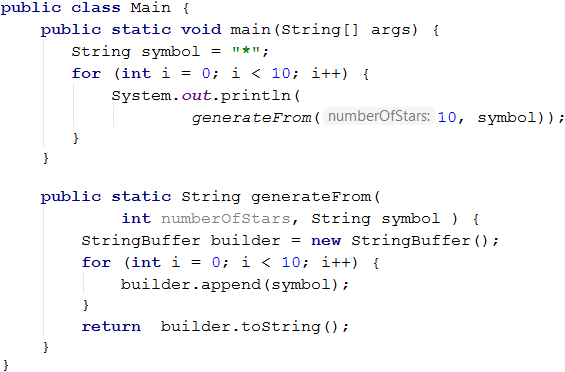How does the example work? The control variable of the loop (i = 0) is initialized. The variable is incremented on each iteration, as long as it is less than 10. After each execution of the code in the loop body, and after the iteration, a check about the state of the variable is performed. Thus the code in the loop body is executed exactly ten times i.e. for each row of the rectangle. In the loop body, we call the method generateFrom. The method uses the class StringBuffer and another for loop. Each iteration of a for loop (the one in the method) will append one asterisk, thus creating a row of ten asterisks. When the whole execution of the loop (the one in the method) finishes, we have a string of asterisks as a result. Then, the execution of the code returns to the point where we call the method. Then, prints the resulting string on the console.

#### Testing in The Judge System

Test your solution here: https://judge.softuni.org/Contests/Practice/Index/657#0.

### Problem: Rectangle of N x N Stars

Write a program that reads an integer n from the console, and as output, draws a rectangle with a size of n x n asterisks.

Input Output Input Output Input Output
2 **
**
3 ***
***
***
4 ****
****
****
****

#### Hints and Guidelines

To perform the problem, we will use the Scanner class, which allows us to read the input size of the figure from the console.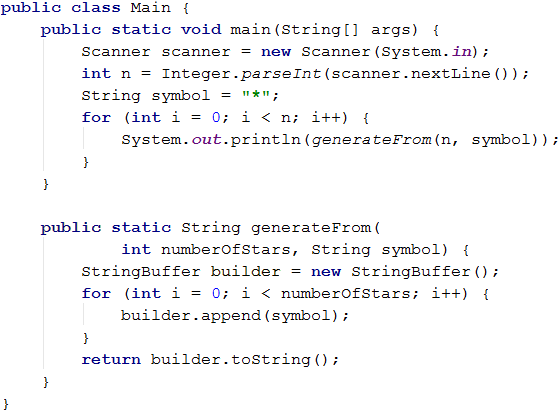#### Testing in The Judge System

Test your solution here: https://judge.softuni.org/Contests/Practice/Index/657#1.

## Nested Loops

Nested loops are construction in which in the body of one loop statement (called outer), another loop statement is performed (called inner). For each iteration of the outer loop statement, the inner one is executed completely. This happens as follows:

• When the execution of nested loops begins, the outer loop starts first which includes the following steps: the control variable is initialized, then checks the condition for the end of the loop, and then the code in the body starts to execute.
• Then the inner loop is executed, which includes the same steps: the control variable is initialized, then checks the condition for the end of the loop, and then the code in the body starts to execute.
• When the condition for the end of the inner loop is met, the program returns one step up, and the started execution of the outer loop continues. This results that the variable of the outer loop is changing (incremented), then checks whether the end condition of the outer loop is satisfied. If not, a new execution of the nested (inner) loop starts.
• This is repeated until the outer loop variable reaches the end of the loop condition.

Here is an example that illustrates the nested loops. The goal is to print a rectangle of n x n asterisks, where for each row, the outer loop iterates from 1 to n, and for each column iterates an inner loop from 1 to n: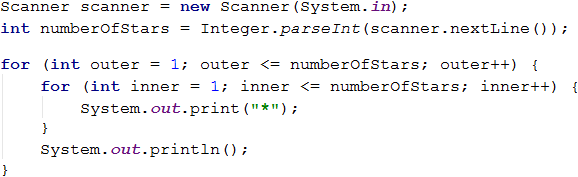How does the above example work? After the initialization of the outer loop, its body begins to execute, which contains another (inner) loop. The inner loop returns numberOfstars as a string of asterisks. After the inner loop completes its execution, the program control returns at the first iteration of the outer loop, and the code within it continues to execute. A statement (System.out.println ()) exists in the body of the outer loop. This statement will move the cursor to the following line when the inner loop completes its execution. Without this code, we will print all asterisks on one line. Usage of println() method in the inner loop instead of the print() method will print all asterisks on separate lines. You can try and see for yourself. Then increment of the variable (in our case by 1) of the outer loop follows, and the whole inner loop starts execution again. The inner loop executes as many times as the body of the outer loop is executed (in our case numberOfstars times).

### Problem: Square of Stars

Write a program that draws on the console a square of N x N asterisks:

Input Output Input Output Input Output
2 * *
* *
3 * * *
* * *
* * *
4 * * * *
* * * *
* * * *
* * * *

#### Hints and Guidelines

The problem is similar to the previous one. Here, it is necessary to consider how to print a space after the asterisks so that there are no unnecessary spaces at the beginning of the end.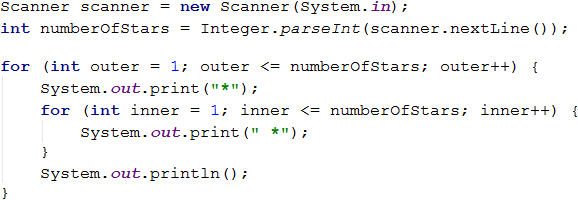#### Testing in The Judge System

Test your solution here: https://judge.softuni.org/Contests/Practice/Index/657#2.

### Problem: Triangle of Dollars

Write a program that reads an integer number n from the console and returns as output a triangle of dollar signs.

Input Output Input Output Input Output
3 $ $ $ $ 
4 $ $ $ $ 
 
5 $ $ $ $ 
 
  \$

#### Hints and Guidelines

The problem is similar to those of drawing rectangles and squares. We will use nested loops again, but there is a trick here. The difference is that the number of columns we need to print as output depends on the row we are on, not the input integer n. From the sample input and output data, we notice that the number of dollars depends on which line we are at the time of printing, i.e. one dollar sign means at the first line, two dollar signs at the second line, etc. Let's look at the example below in more detail. We see that the variable of the nested loop is bound to the variable of the outer loop. In this way, our program prints the desired triangle.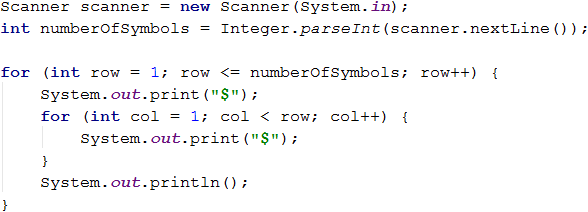#### Testing in The Judge System

Test your solution here: https://judge.softuni.org/Contests/Practice/Index/657#3.

### Problem: Square Frame

Write a program that reads an integer number n from the console and returns as output a square frame with a size of n * n.

Input Output Input Output
3 + - +
| - |
+ - +
4 + - - +
| - - |
| - - |
+ - - +
Input Output Input Output
5 + - - - +
| - - - |
| - - - |
| - - - |
+ - - - +
6 + - - - - +
| - - - - |
| - - - - |
| - - - - |
| - - - - |
+ - - - - +

#### Hints and Guidelines

We can solve the problem as follows:

• We read an integer n from the console.
• We print the upper part of the frame: first the sign +, then n-2 times the sign -, and finally the sign +.
• We print the middle part of the frame: we print n-2 lines as first print the sign |, then n-2 times the sign - and finally again the sign |. We can achieve this with nested loops.
• We print the lower part of the frame: first print the sign +, then n-2 times the sign - and finally the sign +.

Here is an example implementation of the described logic above, with nested loops:#### Testing in The Judge System

Test your solution here: https://judge.softuni.org/Contests/Practice/Index/657#4.

### Problem: Rhombus of Stars

Write a program that reads an integer number n from the console and returns as output a diamond of asterisks with a size of n.

Input Output Input Output
1 * 2  *
* *
 *
Input Output Input Output
3   *
 * *
* * *
 * *
  *
4    *
  * *
 * * *
* * * *
 * * *
  * *
   *

#### Hints and Guidelines

To solve the problem, we divide (imaginary) the rhombus into two parts: upper (includes and the middle row) and lower. We will use two loops for each part of the rhombus to print the output in the console. The reader must find the relationship between n and the variables in the loops.

For the upper part of the rhombus (first loop) we can use the following guidelines:

• We print n-row intervals.
• We print *.
• We print row-1 times *.

We will use the same way to output the lower part of the rhombus, but we leave the reader to try to do it himself.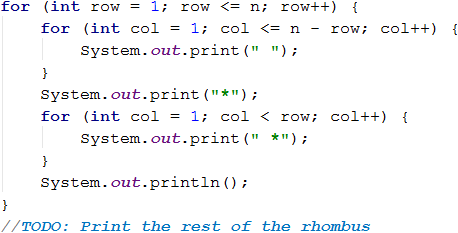#### Testing in The Judge System

Test your solution here: https://judge.softuni.org/Contests/Practice/Index/657#5.

### Problem: Christmas Tree

Write a program that reads an integer number n (1 ≤ n ≤ 100) from the console and returns as output Christmas tree with a height of n+1.

Input Output Input Output
1   |
* | *
2    |
 * | *
** | **
Input Output Input Output
3     |
  * | *
 ** | **
*** | ***
4      |
   * | *
  ** | **
 *** | ***
**** | ****

#### Hints and Guidelines

As we saw in the previous problems, we can apply a similar principle and divide the Christmas tree into three logical parts. The first part is the asterisks (*) and the spaces before and after them, the middle part is |, and the last part are again asterisks (*), and this time empty spaces are only in front of them. Printing the output can be done with one loop, and again we will use the method as we have done at the beginning of this chapter.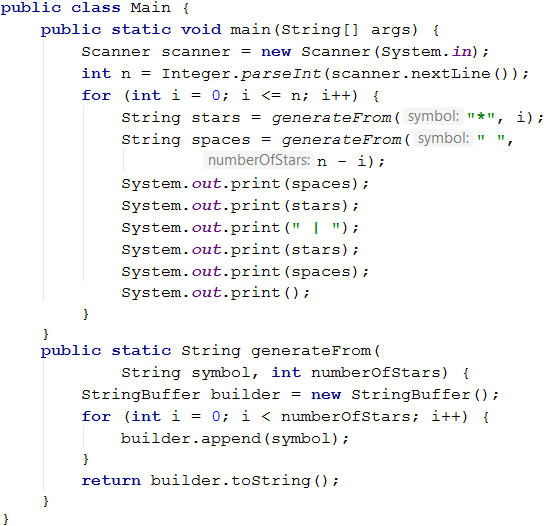#### Testing in The Judge System

Test your solution here: https://judge.softuni.org/Contests/Practice/Index/657#6.

## Drawing More Complex Figures

Let's look at a few exercises about how we can draw figures on the console with more complex logic. To solve these problems, we need to think more about the program logic before we begin writing.

### Problem: Sunglasses

Write a program that reads an integer number n (3 ≤ n ≤ 100) from the console and returns as output sunglasses with a size of 5*n x n, just like the examples below.

Input Output Input Output
3 ******   ******
*////*|||*////*
******   ******
4 ********    ********
*//////*||||*//////*
*//////*    *//////*
********    ********
Input Output
5 **********     **********
*////////*     *////////*
*////////*|||||*////////*
*////////*     *////////*
**********     **********

#### Hints and Guidelines

As we see in the examples below, we can apply a similar principle and divide the sunglasses into three logical parts - upper, middle, and lower. Below is part of the code with which we can solve the problem.

When drawing the top and bottom rows, use 2 * n asterisks, n spaces, and 2 * n asterisks as output.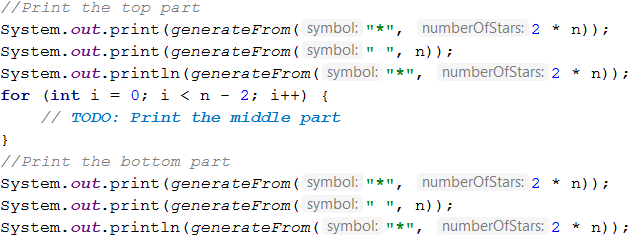When printing the middle part, we need to check whether the line is (n-1) / 2 - 1, because as the example shows on this line, we need to print vertical dashes instead of spaces.#### Testing in The Judge System

Test your solution here: https://judge.softuni.org/Contests/Practice/Index/657#7.

### Problem: House

Write a program that reads an integer number n (2 ≤ n ≤ 100) from the console and returns as output a house with a size of n x n, just like the examples below.

Input Output Input Output Input Output
2 **
||
3 -*-
***
|*|
4 -**-
****
|**|
|**|
Input Output Input Output
5 --*--
-***-
*****
|***|
|***|
8 ---**---
--****--
-******-
********
|******|
|******|
|******|
|******|

#### Hints and Guidelines

According to the condition of the problem, the house has dimensions of n x n, but from the input and output data, we can see the following:

• The house consists of two parts: roof and base.• When n is an even number, the roof of the house is obtuse.
• When n is odd, the roof is one row larger than the base.
##### The Roof
• It consists of asterisks (*) and dashes (-).
• At the top part of the roof, we have one or two stars depending on whether n is even or odd (also related to the dashes).
• In the lowest part, we have many stars and a few or no dashes.
• At each row of the roof (looking from top to bottom), the stars are increased by two, and the dashes are decreased by two.
##### The Base
• The height is n rows.
• It consists of asterisks (*) and pipes (|).
• Each row consists of two pipes (one at the beginning and one at the end of the row) and a sequence of n - 2 asterisks between the pipes.

We read an integer n from the console and assign the value of an integer to a variable of type int.It is of high importance to perform checks on whether the input data is correct! In the current problems, it is not a problem to convert directly the data from the console into int type because it is said that we will read valid integers. If you are making more complex programs, it is a good practice to check the input data.

To draw the roof, we write down how many asterisks we start within a variable called stars:

• If n is even, they are two.
• If n is odd it is one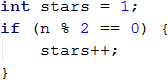We calculate the length of the roof, and it equals half of n. Assign the result to the variable *roofLength.It is important to note that when n is an odd number, the length of the roof is one row bigger than the base one. In Java, when you divide two numbers of integer type with a remainder, the result will be a number without a remainder.

Example:

int result = 3 / 2; // result: 1


If we want to round up the result, we need to use the method Math.ceil(…):

int result = (int) Math.ceil(3 / 2f);


In this example, the division is not between two integers. The letter "f" after a number shows that this number is of float type (a floating-point number). The result of 3 / 2f is 1.5f. Math.ceil(…) method rounds up the result and, in this case, 1.5f will become 2. We write (int) before Math.ceil(…), so we can transfer the result back to int type and assign it to the variable result.

After we have calculated the length of the roof, we use a loop from 0 to roofLength. On each iteration we will:

• Calculate the number of dashes we need to draw. The number will be equal to (n - stars) / 2. Assign it in variable padding.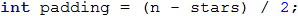• Print on the console: "dashes" (padding times) + "asterisks" (stars times) + "dashes" (padding* times).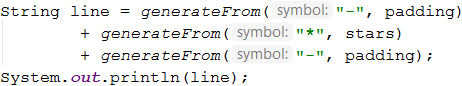• Before the iteration is over, we add 2 to the stars (the number of the asterisks).It is not a good practice to concatenate many character strings as is shown above, because this leads to performance issues. Learn more at: https://docs.oracle.com/javase/7/docs/api/java/lang/StringBuilder.html

After we have finished with the roof, it is time for the base. It is easier to print:

• We use a loop from 0 to n (not inclusive).
• We print on the console: | + * (n - 2 times) + |.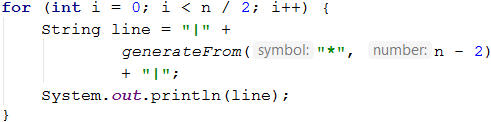#### Testing in The Judge System

Test your solution here: https://judge.softuni.org/Contests/Practice/Index/657#8.

### Problem: Diamond

Write a program that reads an integer number n (1 ≤ n ≤ 100) from the console and returns as output a diamond with a size of n, just like the examples below.

Input Output Input Output Input Output
1 *
2 ** 3 -*-
*-*
-*-
Input Output Input Output Input Output
4 -**-
*--*
-**-
5 --*--
-*-*-
*---*
-*-*-
--*--
6 --**--
-*--*-
*----*
-*--*-
--**--
Input Output Input Output Input Output
7 ---*---
--*-*--
-*---*-
*-----*
-*---*-
--*-*--
---*---
8 ---**---
--*--*--
-*----*-
*------*
-*----*-
--*--*--
---**---
9 ----*----
---*-*---
--*---*--
-*-----*-
*-------*
-*-----*-
--*---*--
---*-*---
----*----

#### Hints and Guidelines

According to the requirements of the problem, have n rows and n columns to draw a diamond, but from the input and output data, we can see the following:

• All lines contain exactly n characters, and all lines, except the upper and the bottom ones, have two asterisks (*).

We can divide (imaginary) the diamond into two parts:

• Upper part. It starts from the top to the middle of the diamond (includes also the middle row).
• Lower part. It starts from the row after the middle up to the bottom tip (inclusive) of the diamond.
##### Upper Part
• If n is odd, begins with 1 asterisk (*).
• If n is even, begins with 2 asterisks (**).
• With each row down, the asterisks move away from each other.
• The space in front, between, and after the asterisks (*) is filled with dashes (-).
##### Lower Part
• With each row down, the asterisks come together. It means that the space (dashes) between them decreases, and the space (dashes) on the left and right increases.
• At its bottom, it has 1 or 2 asterisks, depending on whether n is odd or even.
##### Upper and Lower Part
• On each row, the asterisks are surrounded from the outer side (the left or the right one) by dashes, except the middle row.
• Each row has a space between the two asterisks, except the first and last row (sometimes the asterisk is one).
##### Reading The Input Data

We read an integer n from the console and assign the value of an integer to a variable of type int.##### Printing The Upper Part of The Diamond

We begin drawing the upper part of the diamond. The first thing we need to do is to calculate the number of the outer dashes leftRight (the dashes on the outer side of the asterisks). It is equal to (n - 1) / 2, rounded downward.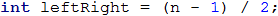After we have calculated leftRight, we start drawing the upper part of the diamond. We can use a loop from 0* to n / 2 + 1** (rounded downward).

At each iteration of the loop the following steps are performed:

• We draw as an output on the console dashes on the left side of the diamond (with length equal to leftRight) and right after them the first asterisk.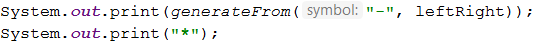• We will calculate the distance between the two asterisks. We can do this by subtracting from n the number of the outer dashes and the number 2 (the number of the asterisks, i.e. the diamond's outline). The result of the subtraction is assigned to a variable mid.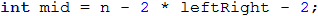• If the mid is lower than 0, we know that on the row should be only 1 asterisk. If it is higher or equal to 0 then we have to print dashes with length equal to mid and one asterisk after them.
• We draw as an output on the console dashes on the right side of the diamond with a length equal to leftRight.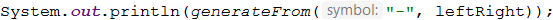• At the end of the loop, we decrease leftRight by 1 (the asterisks * are moving away from each other).

We are ready with the upper part.

##### Printing The Lower Part of The Diamond

Printing the lower part is similar to that of the upper part. The differences are:

• Increase instead of decrease leftRight with one, but at the beginning of the loop
• The loop will be from 0 to (n - 1) / 2.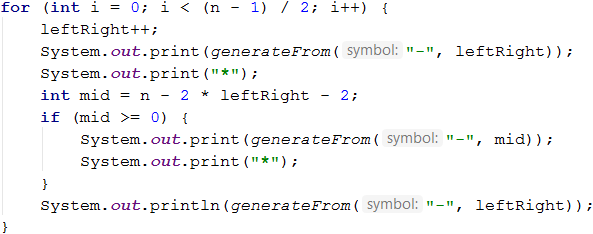Repeating a code is considered bad practice because the code becomes very hard to maintain. Let's imagine that we have a piece of code (e.g. the logic for drawing a row from the diamond example) at a few more places and we decide to change it. For this, we will have to go through all the places and change it everywhere. Now let's imagine that you need to reuse a piece of code not 1, 2, or 3 times but tens of times. A way to overcome this problem is to use methods. You can look for additional information for methods on the Internet or look at Chapter “10” (Methods).

#### Testing in The Judge System

Test your solution here: https://judge.softuni.org/Contests/Practice/Index/657#9.

## What Have We Learned from This Chapter?

We learned to draw figures with nested for loops:

for (int r = 1; r <= 5; r++)
{
System.out.println("*");
for (int c = 1; c < 5; c++)
System.out.println(" *");
System.out.println();
}


We also learned that we can use methods to avoid repeating the code multiple times.